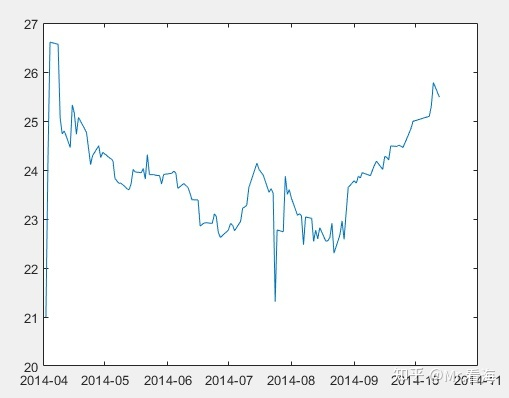# 如何使用优雅地处理时间数据——使用MATLAB以日期为横坐标画图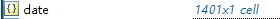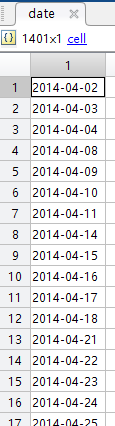``datenn = datenum(date);``

``````plot(datenn,data)
datetick('x','yyyy')``````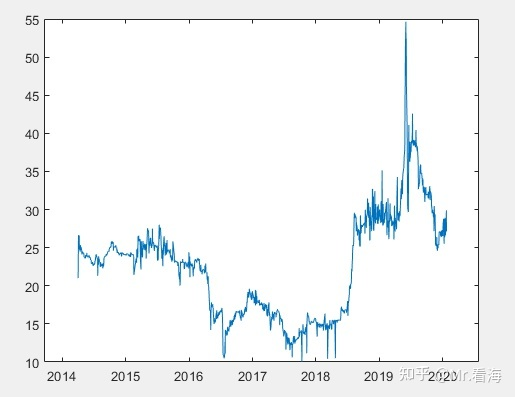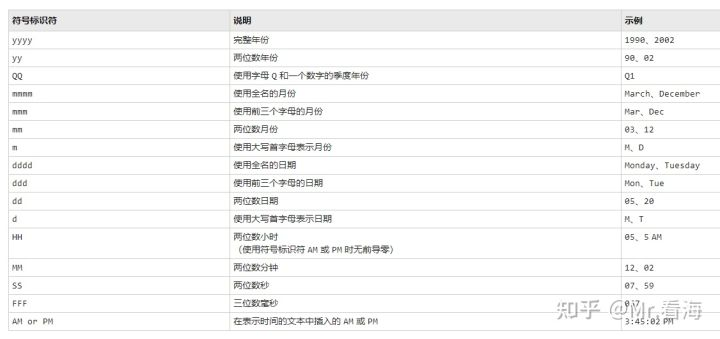``````plot(datenn(1:130),data(1:130))  %为了更好展示效果，截取了部分数据
datetick('x','yyyy-mm')``````